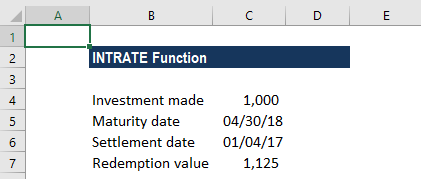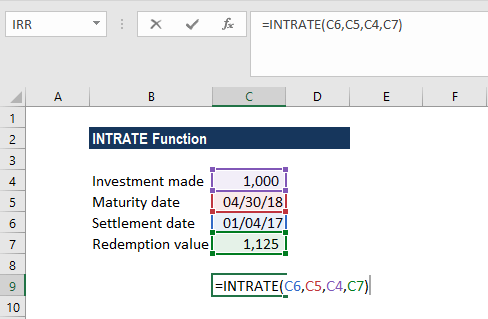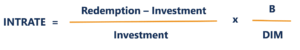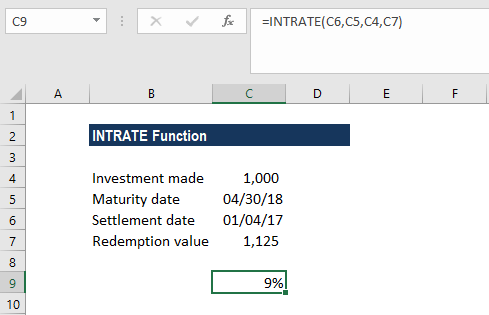# INTRATE Function

Calculates the interest rate for a fully invested security

## What is the INTRATE Function?

The INTRATE Function is categorized under Excel Financial functions. It will calculate the interest rate for a fully invested security. The function was introduced in MS Excel 2007.

As a financial analyst, we regularly come across scenarios where we need to evaluate investments. The INTRATE function is particularly useful in calculating the interest rate of an unlisted bond.

### Formula

=INTRATE(settlement, maturity, investment, redemption, [basis])

The INTRATE function uses the following arguments:

1. Settlement (required argument) – This is the settlement date of the security. The settlement date of a security is the one after the issue date when the security is traded to the buyer.
2. Maturity (required argument) – This is the maturity date of security or the date when the security expires.
3. Investment (required argument) – The amount that is invested in the security.
4. Redemption (required argument) – This is the amount we will receive at maturity.
5. Basis (optional argument) – The type of day count basis to be used. The possible values of the basis are:

BasisDay Count basis
0 or omittedUS(NASD) 30/360
1Actual/actual
2Actual/360
3Actual/365
4European 30/360

### How to use the INTRATE Function in Excel?

As a worksheet function, INTRATE can be entered as part of a formula in a cell of a worksheet. To understand the uses of the function, let us consider an example:

#### Example 1

Let us see the results from this function when we provide the following data:As per the data above, the INTRATE function is used to calculate the interest rate of an investment of \$1,000, which was used to purchase a security on January 4, 2017. The security will mature on April 30, 2018, with a redemption value of \$1,125 and the US (NASD) 30/360 day count basis is used.

The formula to use will be:The formula used in INTRATE calculations is as follows:Where:

• B = number of days in a year, depending on the year basis
• DIM = number of days from settlement to maturity

We get the results below:Remember:

• The date arguments are provided to the function as references to cells containing dates.
• The [basis] argument is omitted so it took the default of 0 (US (NASD) 30/360 basis).

### A few notes about the INTRATE Function:

1. #VALUE! error – Occurs when:
1. The settlement dates given in the argument is not in a valid format.
2. The maturity date is not given in a valid format.
3. Any of the arguments provided is non-numeric.
2. #NUM! error – Occurs when:
1. The given value of the investment is less than or equal to 0.
2. The given redemption value is less than or equal to 0.
3. The given [basis] argument is not equal to 0, 1, 2, 3, or 4.
4. The given maturity date is less than or equal to the supplied settlement date.
3. Remember that Excel stores dates as sequential serial numbers so they can be used in calculations. By default, January 1, 1900 is serial number 1, and January 1, 2018 is serial number 43,101, because it is 43,101 days after January 1, 1900.
4. Settlement, maturity, and basis are truncated to integers if they are in decimal format.

Thanks for reading CFI’s guide to important Excel functions! By taking the time to learn and master these functions, you’ll significantly speed up your financial modeling analysis. To learn more, check out these additional CFI resources:

• Financial Modeling Best Practices